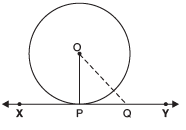Courses

# Facts That Matter- Circles Class 10 Notes | EduRev

## Class 10 : Facts That Matter- Circles Class 10 Notes | EduRev

The document Facts That Matter- Circles Class 10 Notes | EduRev is a part of the Class 10 Course Class 10 Mathematics by VP Classes.
All you need of Class 10 at this link: Class 10

Tangent to a Circle
A tangent to a circle is a line that touches the circle at only one point.

NOTE:
I. There is only one tangent at a point of the circle.
II. The tangent to a circle is a special case of the secant, when the two end points of its corresponding chord coincide.

Theorem 1
The tangent at any point of a circle is perpendicular to the radius, through the point of contact.
Proof: We have the centre O of the given circle and XY is the tangent to the circle at a point P.
Let us take a point Q on XY other than P. Join OQ.
Obviously, Q lies outside the circle. i.e., OQ > OPSince, all the points on XY, except P lies outside the circle.
i.e., OP is smaller than all the distance of the point O from XY.
i.e., OP is the smallest distance of O from XY.
i.e., OP ⊥ XY

Offer running on EduRev: Apply code STAYHOME200 to get INR 200 off on our premium plan EduRev Infinity!

132 docs

,

,

,

,

,

,

,

,

,

,

,

,

,

,

,

,

,

,

,

,

,

;